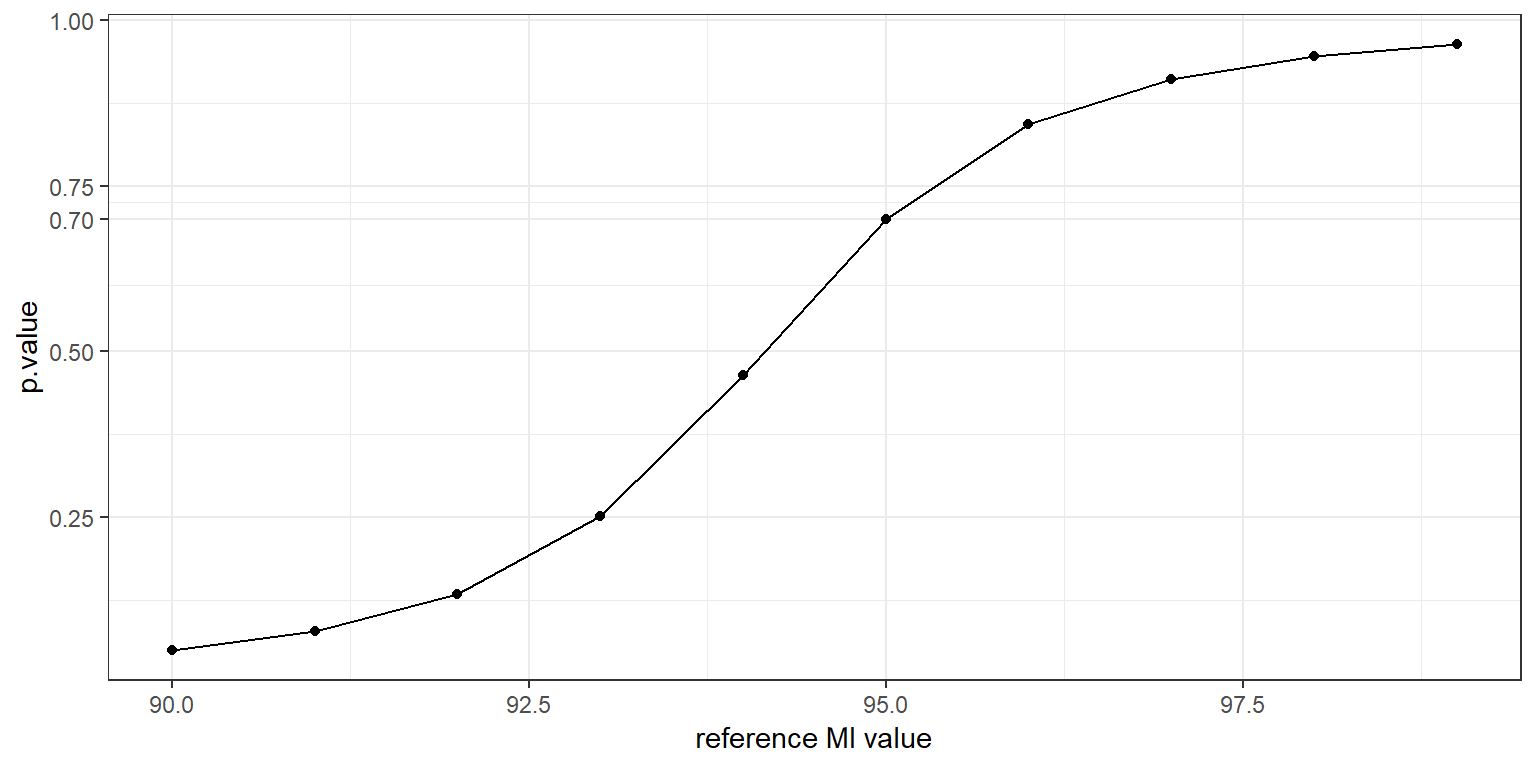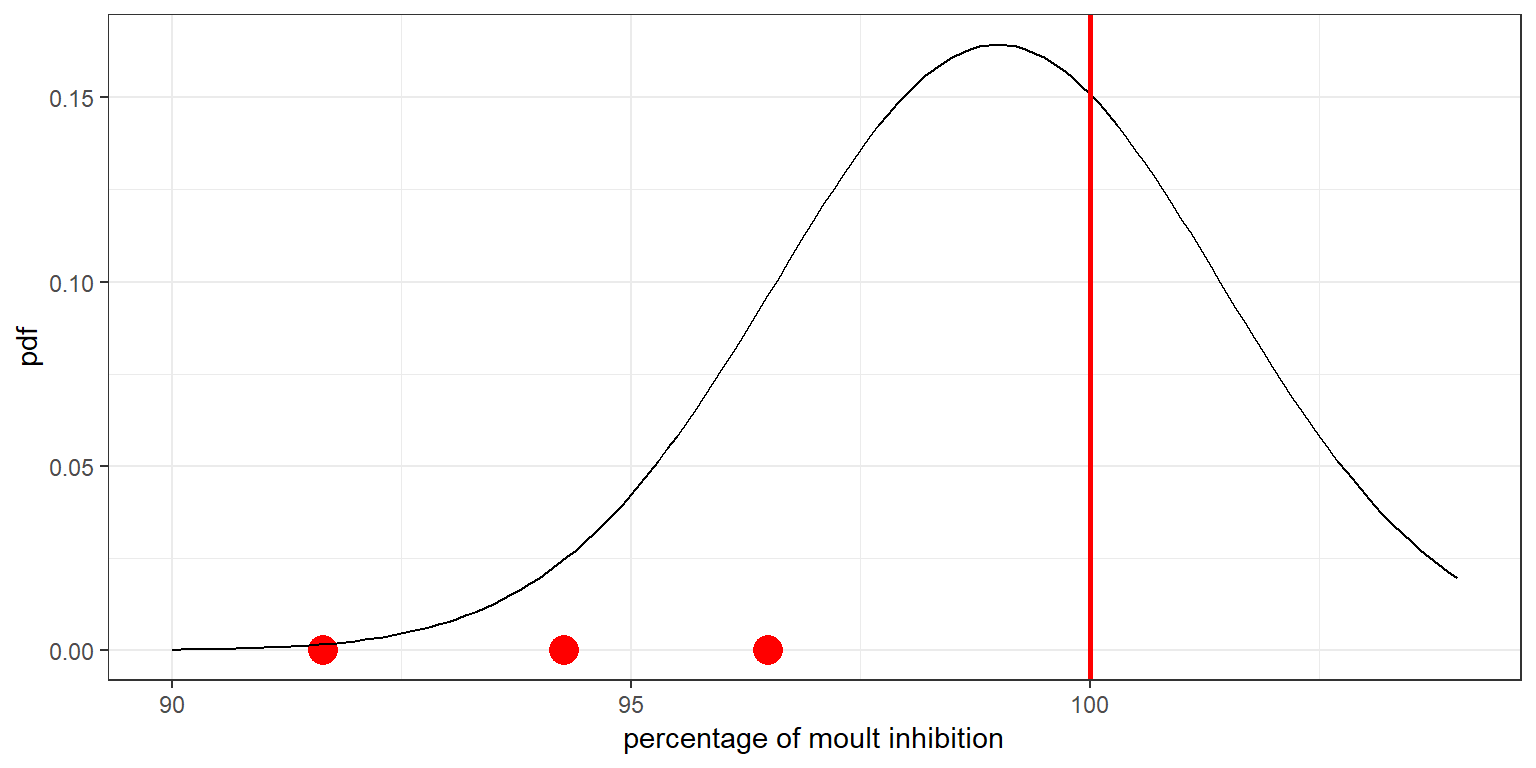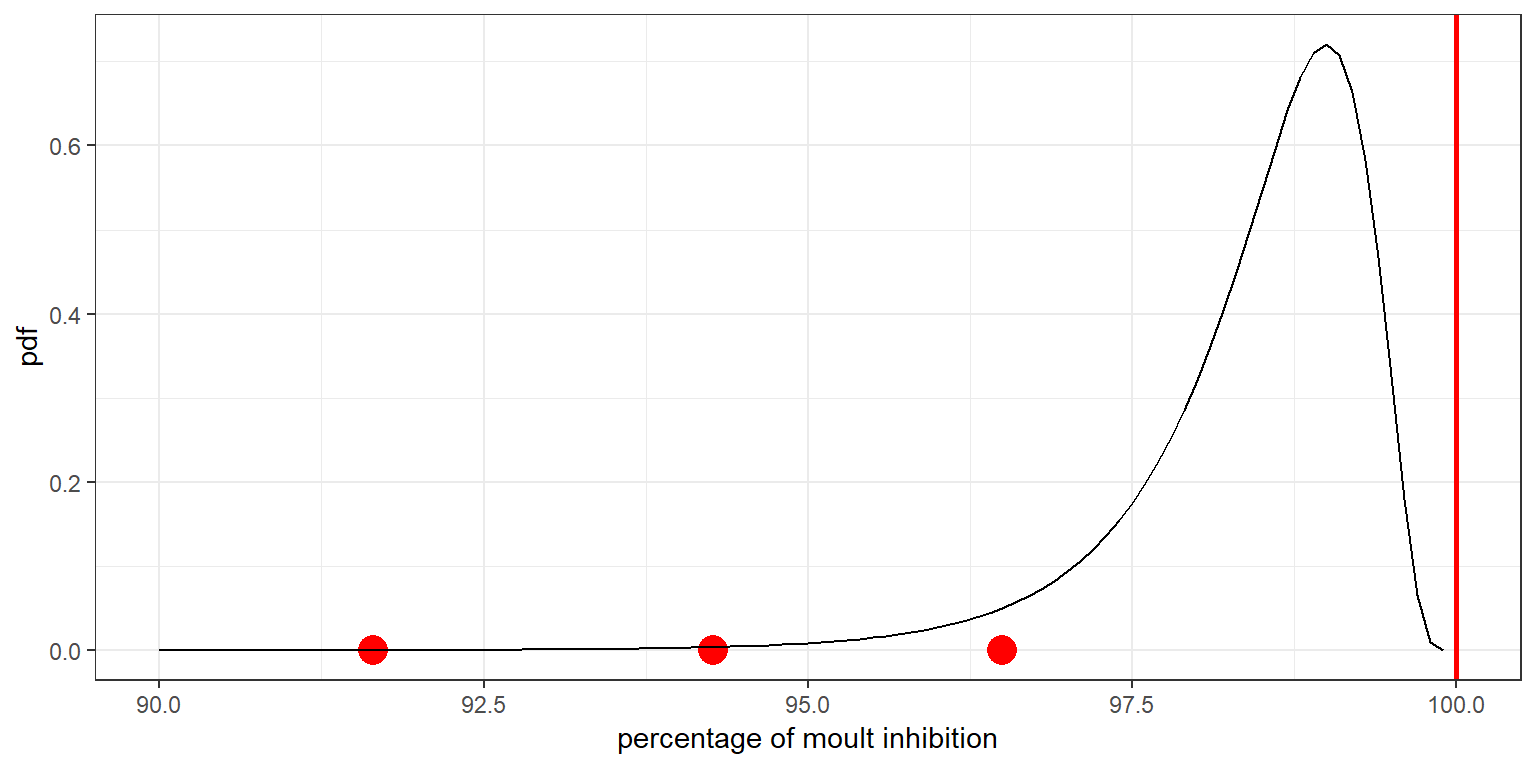$$\newcommand{\esp}{\mathbb{E}\left(#1\right)} \newcommand{\var}{\mbox{Var}\left(#1\right)} \newcommand{\deriv}{\dot{#1}(t)} \newcommand{\prob}{ \mathbb{P}\!(#1)} \newcommand{\eqdef}{\mathop{=}\limits^{\mathrm{def}}} \newcommand{\by}{\boldsymbol{y}} \newcommand{\bc}{\boldsymbol{c}} \newcommand{\bpsi}{\boldsymbol{\psi}} \def\pmacro{\texttt{p}} \def\like{{\cal L}} \def\llike{{\cal LL}} \def\logit{{\rm logit}} \def\probit{{\rm probit}} \def\one{{\rm 1\!I}} \def\iid{\mathop{\sim}_{\rm i.i.d.}} \def\simh0{\mathop{\sim}_{H_0}} \def\df{\texttt{df}} \def\res{e} \def\xomega{x} \newcommand{\argmin}{{\rm arg}\min_{#1}} \newcommand{\argmax}{{\rm arg}\max_{#1}} \newcommand{\Rset}{\mbox{\mathbb{R}}} \def\param{\theta} \def\setparam{\Theta} \def\xnew{x_{\rm new}} \def\fnew{f_{\rm new}} \def\ynew{y_{\rm new}} \def\nnew{n_{\rm new}} \def\enew{e_{\rm new}} \def\Xnew{X_{\rm new}} \def\hfnew{\widehat{\fnew}} \def\degree{m} \def\nbeta{d} \newcommand{\limite}{\mathop{\longrightarrow}\limits_{#1}} \def\ka{k{a}} \def\ska{k{\scriptscriptstyle a}} \def\kel{k{e}} \def\skel{k{\scriptscriptstyle e}} \def\cl{C{\small l}} \def\Tlag{T\hspace{-0.1em}{lag}} \def\sTlag{T\hspace{-0.07em}{\scriptscriptstyle lag}} \def\Tk{T\hspace{-0.1em}{k0}} \def\sTk{T\hspace{-0.07em}{\scriptscriptstyle k0}} \def\thalf{t{1/2}} \newcommand{\Dphi}{\partial_\pphi #1} \def\asigma{a} \def\pphi{\psi} \newcommand{\stheta}{{\theta^\star}} \newcommand{\htheta}{{\widehat{\theta}}}$$

Abstract

According to Monsanto, one of the available weight-of-evidence continuing to support the safety of MON 810 is the absence of demonstrated field resistance for the targeted pests. This conclusion is essentially based on a comparison of 3 observed percentages (91.65%, 96.50%, 94.27%) that measure the susceptibility of insect larvae to a treatment dose that is supposed to inhibit moulting in at least 99% of these larvae. Despite the fact that these 3 values are well below the expected 99%, Monsanto’s statistical analysis pretends to show that these differences are not statistically significant. Unfortunately, this analysis suffers from several flaws (choice of statistical test, choice of null hypothesis) that invalidate the Monsanto findings. This example is quite illustrative of what a more than questionable use of statistics makes it possible to make data say…

# 1 Introduction

## 1.1 Objective of the study

Maize containing event MON 810 is transgenic modified maize expressing the Cry1Ab protein and conferring protection against certain lepidopteran insect pests such as Ostrinia nubilalis and Sesamia nonagrioides.

Resistance development in targeted lepidopteran pests is a potential concern arising from the widespread cultivation of MON 810 maize varieties.

Monsanto established an insect resistance monitoring program in areas where MON 810 is cultivated. The objective is to detect the potential development of resistance that could result in inadequate protection against the target species.

The results of the monitoring plan for S. nonagrioides are presented in a report prepared by Monsanto and available here.

Note that this document is part of the annual monitoring report on the cultivation of MON 810 in 2017 submitted by Monsanto to the European Commission. This report was sent to European Food Safety Authority (EFSA) for an analysis of the results.

## 1.2 Diagnostic concentration bioassays

A diagnostic concentration (DC) of 1091 ng Cry1Ab/cm2, calculated with data from larvae collected in NE Spain over the seasons 2009, 2011, 2013 and 2015, was used for DC bioassays to measure susceptibility to the Cry1Ab protein.

This DC is intended to cause moulting inhibition between 99 and 100% to first instar larvae of S. nonagrioides.

The susceptibility to the protein Cry1Ab by the use of DC bioassays was tested on first filial (F1) progeny of the field populations collected from three different zones in NE Spain in 2017 and on the reference laboratory strain of S. nonagrioides. Moulting inhibition, i.e. percentage of larvae that have not reached the second larval instar, was recorded after 7 days.

## 1.3 Results of the DC bioassays

The average percentage of moulting inhibition (MI) of neonates after treatment at the diagnostic concentration (DC) was estimated for the three three different field populations and the laboratory population:

D <- data.frame(N.control = c(175, 159, 160, 157),
N.treatment   = c(1048, 1111, 1174, 654),
MI.control = c(1.71, 15.09, 6.25, 14.01),
MI.treatment  = c(91.79, 97.03, 94.63, 98.01))
row.names(D) <- c("zone.1", "zone.2", "zone.3", "laboratory")
print(D)
##            N.control N.treatment MI.control MI.treatment
## zone.1           175        1048       1.71        91.79
## zone.2           159        1111      15.09        97.03
## zone.3           160        1174       6.25        94.63
## laboratory       157         654      14.01        98.01
• N.treatment and N.control are, respectively, the numbers of larvae treated in bioassays and the numbers of control larvae
• MI.treatment and MI.control are, respectively, the moulting inhibitions in the treatment group and the control group.

Moulting inhibition values of each zone have been corrected with Abbottâ€™s formula (Abbott, 1925).

$MIc = \left( 1 - \frac{100 - MI_{\rm treatment}}{100 - MI_{\rm control}} \right)\times 100$

D['MIc'] <- round((1 - (1-D$MI.treatment/100)/(1-D$MI.control/100))*100,2)
print(D['MIc'])
##              MIc
## zone.1     91.65
## zone.2     96.50
## zone.3     94.27
## laboratory 97.69

## 1.4 Statistical analysis by Monsanto

Monsanto proposes to use a t-test to compare the average of the 3 (corrected) MI values (91.65%, 96.50%, 94.27%) obtained in the 3 zones with the expected value of 99%. A one-sided test is used to test if the moulting inhibition mean has decreased ($$H_0: \ \mu \geq 99$$ vs $$H_1: \ \mu < 99$$):

D.field <- D[c('zone.1', 'zone.2', 'zone.3'),]
MIc.field <- D.field[['MIc']]
MI.ref <- 99
t.test(MIc.field, mu=MI.ref, alternative ="less")
##
##  One Sample t-test
##
## data:  MIc.field
## t = -3.4675, df = 2, p-value = 0.03702
## alternative hypothesis: true mean is less than 99
## 95 percent confidence interval:
##     -Inf 98.2326
## sample estimates:
## mean of x
##     94.14

Monsanto also performed a t-test to determine if the observed percentage of moulting inhibition was significantly lower than the percentage of moulting inhibition observed in the susceptible reference strain after treatment at the same DC

MIc.lab <- D['laboratory','MIc']
t.test(MIc.field, mu=MIc.lab, alternative ="less")
##
##  One Sample t-test
##
## data:  MIc.field
## t = -2.5329, df = 2, p-value = 0.06344
## alternative hypothesis: true mean is less than 97.69
## 95 percent confidence interval:
##     -Inf 98.2326
## sample estimates:
## mean of x
##     94.14

These results are summarized and commented pp~11-12 of the Insect Resistance Monitoring Report - 2017:

Of the total F1 neonates originated from the field collected larvae, 3333 were used in the bioassays. The DC (1091 ng Cry1Ab/cm2) caused a mean (Â± S.E.) moulting inhibition of 94.14% Â± 1.4% (91.65%, 96.50% and 94.28% in larvae from zone 1, zone 2 and zone 3, respectively; Table 8). This value was significantly lower than the expected value of 99% (t = -3.4647, df = 2, p = 0.037). However, the same DC applied to neonates of the laboratory strain of S. nonagrioides caused moulting inhibition of 97.69% (Table 8). In this case, the average value obtained with field F1 neonates (94.14%) was not significantly lower from the moult inhibition value of the reference strain (t = -2.5373, df = 2, p = 0.063).

Our objective here is to explain why the conclusions formulated by Monsanto on the basis of these results are questionable.

# 2 Comments on the statistical analysis

## 2.1 Choice of the null hypothesis

We have seen that a one sided 95% confidence interval for the theoretical mean of moulting inhibition is $$(-\infty, 98.23)$$. In other word, the observed mean moulting inhibition of 94.14% cannot be considered as significantly lower than any value of this interval. And even then, this value is almost not significantly lower than the expected value of 99%.

But can we conclude from this that the susceptibility to the Bt toxin has not decreased? Of course no!

Indeed, to simply state that the null hypothesis ($$\mu \geq 97.69%$$, for instance) cannot be rejected is not informative enough. In particular, the choice of the null hypothesis is critical. Monsanto takes as a reference hypothesis the fact that there is no attenuation in inhibition with respect to the reference strain: $H_0 \ : \mu \geq 97.69\% " \quad vs \quad H_1 \ : \mu < 97.69\% "$ It is then up to the data to demonstrate the opposite.

But given the very small number of data (3), it is necessary to observe a very large decrease to make it statistically significant. In our example, even if the null hypothesis is quite unlikely ($$p=0.063$$), it cannot be formally rejected if we choose a significance level of 5%.

But what happens if we now choose to define as a null hypothesis the fact that resistance has increased in a biologically significant way?

Imagine for instance that an environmental organization claims that the expected percentage of moulting inhibition is less that 91%. Do the same data allow to demonstrate the contrary?

The hypotheses of the test are now: $H_0 \ : \mu \leq 91\% " \quad {\rm vs} \quad H_1 \ : \mu > 91\% "$

It can then be seen that the data collected in 2017 do not allow this hypothesis to be rejected ($$p=0.077$$):

t.test(MIc.field, mu=91, alternative ="greater")
##
##  One Sample t-test
##
## data:  MIc.field
## t = 2.2403, df = 2, p-value = 0.07719
## alternative hypothesis: true mean is greater than 91
## 95 percent confidence interval:
##  90.0474     Inf
## sample estimates:
## mean of x
##     94.14

The graph below displays the $$p$$-values of the test $$\mu \leq \mu_0$$ vs $$\mu > \mu_0$$ for several values of $$\mu_0$$:

R <- data.frame(ref=90:99)
R$p.value <- 1-pt((mean(MIc.field)- R$ref)/sd(MIc.field)*sqrt(3),2)
ggplot(R, aes(ref,p.value)) + geom_line() + geom_point() + xlab("reference MI value") + scale_y_continuous(breaks=c(0.25, 0.50, 0.70, 0.75, 1))In particular, it can be seen that it is very likely that the expected percentage of moulting inhibition of neonates after treatment at the diagnostic concentration is less than 95% (p=0.70), which indicates a multiplication by at least 5 of the resistance of S. nonagrioides larvae to diagnostic concentration.

## 2.2 Choice of the statistical test

The use of a t-test assumes that the data are (roughly) normally distributed. In particular, under the null hypothesis $$\mu=99\%$$, the moult inhibition values obtained in the different zones are assumed to be normally distributed around $$99\%$$:

x <- seq(90,104,by=0.1)
sd.MIc <- sd(MIc.field)
r <- data.frame(x=x, y=dnorm(x, MI.ref, sd.MIc))
ggplot(D.field) + geom_point(aes(x=MIc,y=0), color="red", size=5) +
geom_line(data=r, aes(x,y)) + geom_vline(xintercept = 100, colour='red', size=1)  + ylab('pdf') + xlab("percentage of moult inhibition")The graph shows very well that the normal distribution is not at all suitable for these data. Indeed, this normal distribution is symmetrical around 99%, which makes no sense since the values recorded are necessarily less than 100%.

One way to make data normal is by applying a well-chosen transformation to it. A logit ype transformation is well suited when the data $$x_1, x_2, \ldots, x_n$$ takes its values in a bounded interval such as $$(0, 100)$$: $\tilde{x}_i = \log \left( \frac{x_i}{100-x_i} \right)$ Then, the transformed variable $$\tilde{x}_i$$ can take any positive or negative values. Assuming that the transformed variables $$(\tilde{x}_i)$$ are normally distributed is equivalent to assuming that the original variables $$(x_i)$$ are logit-normally distributed:

x <- seq(90,99.9, by=0.1)
yt <- dnorm(log(x/(100-x)), log(MI.ref/(100-MI.ref)), sd(log(MIc.field/(100-MIc.field)))*1.2)
r <- data.frame(x=x, y=yt)
ggplot(D.field) + geom_point(aes(x=MIc,y=0), color="red", size=5) +
geom_line(data=r, aes(x,y)) + geom_vline(xintercept = 100, colour='red', size=1)  + ylab('pdf') + xlab("percentage of moult inhibition")The average of the transformed variables $$(\tilde{x}_i, 1 \leq i \leq n)$$ can now be compared to the transformed reference values $$\log(99/(100-99))$$ and $$\log(97.69/(100-97.69))$$

t.test(log(MIc.field/(100-MIc.field)), mu=log(MI.ref/(100-MI.ref)), alternative ="less")
##
##  One Sample t-test
##
## data:  log(MIc.field/(100 - MIc.field))
## t = -6.5937, df = 2, p-value = 0.01112
## alternative hypothesis: true mean is less than 4.59512
## 95 percent confidence interval:
##      -Inf 3.615935
## sample estimates:
## mean of x
##  2.837648
t.test(log(MIc.field/(100-MIc.field)), mu=log(MIc.lab/(100-MIc.lab)), alternative ="less")
##
##  One Sample t-test
##
## data:  log(MIc.field/(100 - MIc.field))
## t = -3.4025, df = 2, p-value = 0.03829
## alternative hypothesis: true mean is less than 3.744552
## 95 percent confidence interval:
##      -Inf 3.615935
## sample estimates:
## mean of x
##  2.837648

Thus, a correct use of the t-test for these data shows that the average percentage of moulting inhibition of neonates after treatment at the diagnostic concentration is:

• significantly lower than the expected generical value of 99% ($$p=0.011$$),
• significantly lower than the percentage of moulting inhibition observed in the susceptible reference strain ($$p=0.038$$).

## 2.3 Individual comparisons

Beyond the problems raised by the choice of statistical test and the choice of hypothesis to be tested, the general approach used by Monsanto to analyze these data is highly questionable. Indeed, each percentage obtained in a zone is considered as a random variable for which only the mean value is of interest.

However, the three observed percentages of moulting inhibition (91.65%, 96.50%, 94.27%), corresponding to 3 different geographical zones, were obtained on large samples (larger than 1000) and should be analyzed individually.

Let $$\mu_1$$, $$\mu_2$$ and $$\mu_3$$ be the three expected percentages of moulting inhibition for these 3 zones.

Exact Fisher tests show that these three expected percentages are different:

N.zone1 <- round(D.field$N.treatment*c(MIc.field, 100-MIc.field)/100) N.zone2 <- round(D.field$N.treatment*c(MIc.field, 100-MIc.field)/100)
N.zone3 <- round(D.field$N.treatment*c(MIc.field, 100-MIc.field)/100) p12 <- fisher.test(matrix(c(N.zone1,N.zone2),nrow=2))$p.value
p13 <- fisher.test(matrix(c(N.zone1,N.zone3),nrow=2))$p.value p23 <- fisher.test(matrix(c(N.zone2,N.zone3),nrow=2))$p.value
P <- data.frame(p.value=signif(c(p12,p13,p23),3), row.names=c("zone.1 vs zone.2", "zone.1 vs zone.3","zone.2 vs zone.3") )
print(P)
##                   p.value
## zone.1 vs zone.2 1.45e-06
## zone.1 vs zone.3 1.54e-02
## zone.2 vs zone.3 1.29e-02

There is therefore a significant inter-zone variability in term of moulting inhibition of neonates after treatment at the diagnostic concentration.

95% confidence intervals for the three different unknwon percentages $$\mu_1$$, $$\mu_2$$ and $$\mu_3$$ can be computed:

D.field$sd <- sqrt(D.field$MIc*(100-D.field$MIc)/D.field$N.treatment)
D.field$CI.lower <- D.field$MIc+qnorm(0.025)*D.field$sd D.field$CI.upper <- D.field$MIc+qnorm(0.975)*D.field$sd
print(D.field[c('CI.lower', 'CI.upper')])
##        CI.lower CI.upper
## zone.1 89.97515 93.32485
## zone.2 95.41934 97.58066
## zone.3 92.94053 95.59947

Neither the theoretical value of 99% nor the value obtained from the reference stain (97.69%) belong to any of these confidence intervals. Then, the hypothesis that $$\mu_1 \geq 99%$$ (resp. $$\mu_2 \geq 99%$$ and $$\mu_3 \geq 99%$$) is rejected without any hesitation:

sd.ref <- sqrt(MI.ref*(100-MI.ref)/D.field$N.treatment) D.field$p.ref <- pnorm(D.field$MIc, MI.ref, sd.ref) print(D.field['p.ref']) ## p.ref ## zone.1 1.097802e-126 ## zone.2 2.763625e-17 ## zone.3 5.968548e-60 We show in the same way that $$\mu_1<97.69%$$, $$\mu_2<97.69%$$ and $$\mu_3<97.69%$$: sd.lab <- sqrt(MIc.lab*(100-MIc.lab)/D.field$N.treatment)
D.field$p.lab <- pnorm(D.field$MIc, MIc.lab, sd.lab)
print(D.field['p.lab'])
##               p.lab
## zone.1 4.944250e-39
## zone.2 4.140172e-03
## zone.3 3.080193e-15

It can therefore be concluded that there was a significant reduction in moulting inhibition in 2017 in each of the three zones from Northeast of Spain.